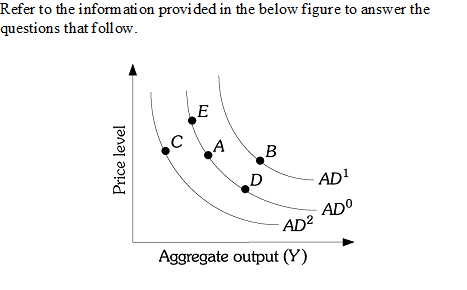### Create an Account

Home / Questions / efer to graph 3. Assume the central bank decreases the money supply. This can be illustrat...

# efer to graph 3. Assume the central bank decreases the money supply. This can be illustrated by * A movement from point E to A A movement from Point D to E A shift in the Aggregate demand from

efer to graph 3. Assume the central bank decreases the money supply. This can be illustrated by *

A movement from point E to A

A movement from Point D to E

2-Refer to graph 3. If the required reserve ratio decreases , then : *

Aggregate demand curve shifts from AD0 to AD1, Real GDP and Price level decreases

Aggregate demand curve shifts from AD0 to AD1, Real GDP and Price level increases

Aggregate demand curve sifts from AD0 to AD2, Real GDP and price level increases

Aggregate demand curve sifts from AD0 to AD2, Real GDP and price level decreasesApr 15 2021 View more View LessSubscribe To Get Solution